# NR Cell Performance Evaluation with Beam Management

This example models a 5G New Radio (NR) cell with transmit-end beam refinement procedure in the downlink (DL) direction and evaluates the network performance. This example shows how to transmit multiple single-port channel state information reference signal (CSI-RS) resources in different directions for Layer 1 (physical layer) reference signal received power (L1-RSRP) measurements. The gNB scheduler uses the L1-RSRP reports to beamform the physical downlink shared channel (PDSCH) transmissions for better signal-to-noise-ratio (SNR) at the UEs. You can modify the gNB scheduler to select the beam direction for PDSCH transmissions.

### Introduction

Downlink beamforming improves the network performance by utilising the multi-antenna configuration to focus energy of the signals in a direction which results in better SNR at a UE. Additionally, beamforming also limits the interference in other directions. For efficient DL beamforming, gNB relies on the channel state information resource indicator (CRI) and L1-RSRP feedback from the UEs. The UEs measure the L1-RSRP on multiple CSI-RS resources where each resource corresponds to one direction, and reports the gNB with the CRI having the highest L1-RSRP.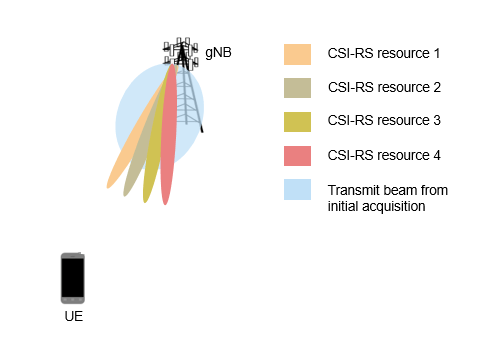The example models multiple sets of CSI-RS beams for L1-RSRP measurements. Each set corresponds to a wider synchronization signal block (SSB) beam and contains finer CSI-RS beams within the SSB beam. The example does not model the SSB beam sweeping process for initial acquisition, and assumes the SSB beam associated with each UE to be the beam with angular range covering the line-of-sight path from the gNB.

This example models:

• DL channel quality measurement and reporting by UEs based on the multi-port CSI-RS received from the gNB. The report includes rank indicator (RI), precoding matrix indicator (PMI), and channel quality indicator (CQI). The example supports Type-1 single-panel codebook for PMI.

• DL L1-RSRP measurement and CRI reporting by UEs based on the single-port CSI-RS received from the gNB.

• Transmit end beam refinement in DL direction using CRI-RSRP feedback.

• Single-codeword DL spatial multiplexing to perform multi-layer transmission. Single-codeword limits the number of transmission layers to 4.

• Precoding to map the transmission layers to antenna ports.

• Digital beamforming of PDSCH based on the CRI feedback from the UEs.

• Free space path loss (FSPL), additive white Gaussian noise (AWGN), and clustered delay line (CDL) propagation channel model.

Nodes send the control packets (buffer status report (BSR), DL assignment, UL grants, PDSCH feedback, CSI report, and CRI-RSRP reports) out of band, without the need of resources for transmission and assured error-free reception.

### MIMO

The key aspects of multiple-input multiple-output (MIMO) include precoding, beamforming, channel measurement and reporting.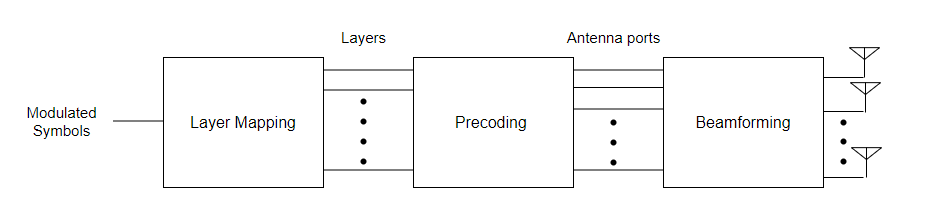#### Layer mapping

The layer mapping process maps the modulated symbols of the codeword onto different layers.

#### Precoding

Precoding, which follows the layer mapping, maps the transmission layers to antenna ports. Precoding applies a precoding matrix to the transmission layers and outputs data streams to the antenna ports.

#### Beamforming

Beamforming involves the use of multiple radiating elements transmitting the same signal to produce a longer and narrower beam in a particular direction. The higher the number of antennas, the narrower the beamwidth.

Beam management is a set of physical (PHY) layer and medium access control (MAC) layer procedures to acquire and maintain a set of beam pair links (a beam used at gNB paired with a beam used at UE). There are three procedures defined for beam management. For more detailed information about the beam management procedure, refer to NR Downlink Transmit-End Beam Refinement Using CSI-RS example.

The current example focuses on the Procedure 2 (P-2) beam measurement and beam reporting procedures which involves sending single port CSI-RS resources over highly directional beams to each UE. The L1-RSRP measurements over each of these resources are then reported back to the gNB which is used to perform transmit beam refinement for all subsequent downlink transmissions intended for the UE till the next L1-RSRP measurement.

#### DL Channel Measurement and Reporting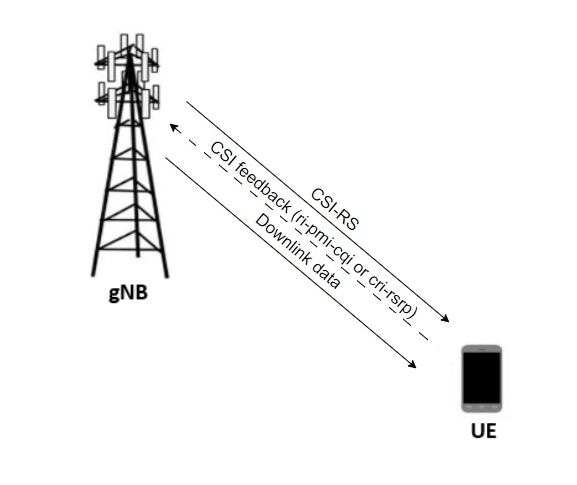CSI reporting is the process by which a UE, for DL transmissions, advises a suitable number of transmission layers (rank), PMI, and CQI values to the gNB. The UE estimates these values by performing channel measurements on its configured CSI-RS resources. For more details, see the 5G NR Downlink CSI Reporting example. The gNB scheduler uses this advice to decide the number of transmission layers, precoding matrix, and modulation and coding scheme (MCS) for PDSCHs.

The UE also uses the CSI report to indicate the CRI, and the L1-RSRP to aid the gNB in transmit beam refinement.

### NR Protocol Stack

A node (gNB or UE) is a composition of NR stack layers. The helper classes hNRGNB.m and hNRUE.m create gNB and UE nodes, respectively, containing the radio link control (RLC), MAC and PHY layer. For more details, see the NR Cell Performance Evaluation with Physical Layer Integration example.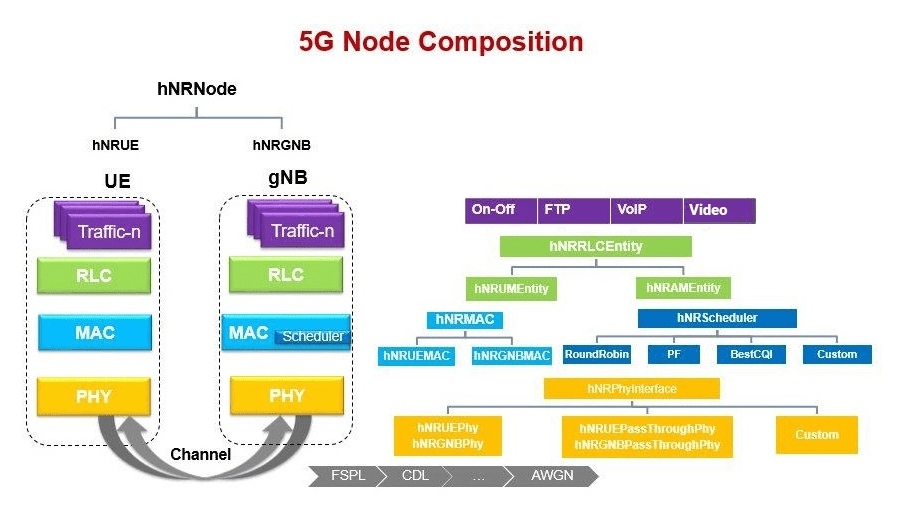### Scenario Configuration

Check if the Communications Toolbox Wireless Network Simulation Library support package is installed. If the support package is not installed, MATLAB® returns an error with a link to download and install the support package.

`wirelessnetworkSupportPackageCheck`

Configure simulation parameters in the `simParameters` structure.

```rng('default'); % Reset the random number generator simParameters = []; % Clear the simParameters variable simParameters.NumFramesSim = 10; % Simulation time in terms of number of 10 ms frames```

Enable or disable DL beamforming.

`enableBeamforming = true;`

Specify the azimuth sweep angles and direction of SSBs.

Azimuth angle is the angle measured in the horizontal plane ranging from [-180, 180] and

Elevation angle is the angle measured in the vertical plane ranging from [-90, 90] between horizontal plane and line of sight (LOS) point in the Spherical coordinate system.

```if enableBeamforming % Number of SSBs numSSBs = 4; % Total azimuthal range for all SSBs corresponding to a 120 degrees sectorized cell azSweepRange = [-60, 60]; validateattributes(azSweepRange,{'numeric'},{'nonempty','real', 'vector', ... '>=',-60,'<=',60,'finite'}, ... 'azSweepRange','azimuthal range'); % Sweep range for non-overlapping SSB directions ssbSweepRange = diff(azSweepRange)/numSSBs; % Azimuthal angles for SSB beam sweeping in the azimuthal range ssbTxAngles = [-60 -20 20 60]; % Elevation range for all SSBs sweeping in the azimuthal angles elSweepRange = [-45, 0]; end```

Specify the number of UEs in the cell, assuming that UEs have sequential radio network temporary identifiers (RNTIs) from `1` to `simParameters.NumUEs`. If you change the number of UEs, ensure that the number of rows in `simParameters.UEPosition` is equal to the value of simParameters.NumUEs.

```simParameters.NumUEs = 3; % Position of gNB in (x,y,z) coordinates simParameters.GNBPosition = [0, 0, 0]; % Specify UE position in spherical coordinates (r,azimuth,elevation) % relative to gNB. Here 'r' is the distance, 'azimuth' is the angle in the % horizontal plane and elevation is the angle in the vertical plane. When % beamforming is enabled, all the UE positions must lie with in the azimuth % and elevation range limits of SSBs as defined above. It is a matrix of % size N-by-3 where N is the number of UEs. Row 'i' of the matrix contains % the coordinates of UE with RNTI 'i' ueRelPosition = [500, -5, -5; 500, -60, -30; 500, -10, -20]; if enableBeamforming validateattributes(ueRelPosition(:,2),{'numeric'},{'nonempty','real','vector', ... '>=',azSweepRange(1),'<=',azSweepRange(2),'finite'}, ... 'ueRelPosition(:,2)','azimuth angles'); validateattributes(ueRelPosition(:,3),{'numeric'},{'nonempty','real','vector', ... '>=',elSweepRange(1),'<=',elSweepRange(2),'finite'}, ... 'ueRelPosition(:,3)','elevation angles'); end % Convert Spherical to Cartesian coordinates considering gNB position as origin [xPos, yPos, zPos] = sph2cart(deg2rad(ueRelPosition(:, 2)),deg2rad(ueRelPosition(:, 3)), ... ueRelPosition(:, 1)); % Global coordinates of UEs simParameters.UEPosition = [xPos, yPos, zPos] + simParameters.GNBPosition; % Validate the UE positions validateattributes(simParameters.UEPosition, {'numeric'},{'nonempty','real', ... 'nrows',simParameters.NumUEs,'ncols',3,'finite'}, ... 'simParameters.UEPosition','UEPosition');```

Set the channel bandwidth to 5 MHz and the subcarrier spacing (SCS) to 15 kHz as defined in 3GPP TS 38.104 Section 5.3.2.

```simParameters.NumRBs = 25; simParameters.SCS = 15; % kHz simParameters.DLBandwidth = 5e6; % Hz simParameters.ULBandwidth = 5e6; % Hz simParameters.DLCarrierFreq = 2.646e9; % Hz simParameters.ULCarrierFreq = 2.535e9; % Hz```

Specify the transmit (Tx) and receive (Rx) antenna panel geometry at the gNB and UEs in the format `(M,N,P)`, where `M` and `N` are the number of rows and columns in the antenna array, respectively. `P` is the number of polarizations (1 or 2).

```gNBTxArraySize = [2 4 2]; ueRxArraySize = repmat([1 1 2],simParameters.NumUEs,1);```

Configure the gNB transmit antenna panel.

```lambda = physconst('LightSpeed')/simParameters.DLCarrierFreq; simParameters.TxAntPanel = phased.NRRectangularPanelArray('ElementSet', ... repmat({phased.NRAntennaElement},1,gNBTxArraySize(3)), ... 'Size',[gNBTxArraySize(1:2) 1 1],'Spacing', ... [0.5*lambda 0.5*lambda 1 1]);```

Specify the CSI-RS configuration for RI, PMI and CQI measurements.

```csirs = cell(1,simParameters.NumUEs); csirsOffset = [5 6 10 11]; for csirsIdx = 1:simParameters.NumUEs csirs{csirsIdx} = nrCSIRSConfig('NID',1,'NumRB',simParameters.NumRBs, ... 'RowNumber',11,'SubcarrierLocations',[1 3 5 7], ... 'SymbolLocations',0,'CSIRSPeriod',[20 csirsOffset(csirsIdx)]); end simParameters.CSIRSConfig = csirs;```

Specify the CSI-RS resource set configuration associated with each SSB for DL beam refinement. Each resource set contains multiple single-port CSI-RS resources, with each resource corresponding to a particular beam direction within the SSB angular range.

```if enableBeamforming simParameters.NumCSIRSBeams = 4; csirsConfigRSRP = cell(1,numSSBs); for ssbIdx = 1:numSSBs csirsConfig = nrCSIRSConfig; csirsConfig.NID = 1; csirsConfig.CSIRSType = repmat({'nzp'},1,simParameters.NumCSIRSBeams); csirsConfig.CSIRSPeriod = [40 0]; csirsConfig.Density = repmat({'one'},1,simParameters.NumCSIRSBeams); csirsConfig.RowNumber = repmat(2,1,simParameters.NumCSIRSBeams); csirsConfig.SymbolLocations = {1,5,6,7}; csirsConfig.SubcarrierLocations = repmat({ssbIdx-1},1, ... simParameters.NumCSIRSBeams); csirsConfig.NumRB = simParameters.NumRBs; csirsConfigRSRP{ssbIdx} = csirsConfig; end simParameters.CSIRSConfigRSRP = csirsConfigRSRP; end```

Specify the signal-to-interference-plus-noise ratio (SINR) to a CQI index mapping table for a block error rate (BLER) of 0.1. The lookup table corresponds to the CQI table as per 3GPP TS 38.214 Table 5.2.2.1-3.

```simParameters.DownlinkSINR90pc = [-0.4600 4.5400 9.5400 14.0500 16.540 19.0400 ... 20.5400 23.0400 25.0400 27.4300 29.9300 30.4300 ... 32.4300 35.4300 38.4300];```

Specify the gNB transmit power.

`simParameters.GNBTxPower = 34; % In dBm`

Configure the channel model.

```channelModelDL = cell(1,simParameters.NumUEs); waveformInfo = nrOFDMInfo(simParameters.NumRBs,simParameters.SCS); dlchannel = nrCDLChannel('DelayProfile','CDL-D','DelaySpread',300e-9); for ueIdx = 1:simParameters.NumUEs cdl = hMakeCustomCDL(dlchannel); % Configure the channel seed based on the UE number % Independent fading for each UE cdl.Seed = cdl.Seed + (ueIdx - 1); % Compute the LOS angle from gNB to UE [~,depAngle] = rangeangle(simParameters.UEPosition(ueIdx, :)', ... simParameters.GNBPosition'); % Configure the azimuth and zenith angle offsets for this UE cdl.AnglesAoD(:) = cdl.AnglesAoD(:) + depAngle(1); % Convert elevation angle to zenith angle cdl.AnglesZoD(:) = cdl.AnglesZoD(:) - cdl.AnglesZoD(1) + (90 - depAngle(2)); % Compute the range angle from UE to gNB [~,arrAngle] = rangeangle(simParameters.GNBPosition',... simParameters.UEPosition(ueIdx, :)'); % Configure the azimuth and zenith arrival angle offsets for this UE cdl.AnglesAoA(:) = cdl.AnglesAoA(:) - cdl.AnglesAoA(1) + arrAngle(1); % Convert elevation angle to zenith angle cdl.AnglesZoA(:) = cdl.AnglesZoA(:) - cdl.AnglesZoA(1) + (90 - arrAngle(2)); cdl.CarrierFrequency = simParameters.DLCarrierFreq; cdl.TransmitAntennaArray = simParameters.TxAntPanel; cdl.ReceiveAntennaArray.Size = [ueRxArraySize(ueIdx, :) 1 1]; cdl.SampleRate = waveformInfo.SampleRate; channelModelDL{ueIdx} = cdl; end```

Initialize the beamforming weights table for DL beam refinement. It contains the steering vectors corresponding to direction of various CSI-RS beams across all the SSBs. It is of size M x N, where M represents number of transmit antenna elements and N represents number of CSI-RS beams of all SSBs.This table is stored at gNB PHY. For each PDSCH, scheduler selects a column index in this table (corresponding to the beam direction) and conveys the same to PHY.

```% Number of transmit antennas numTxAntennas = prod(gNBTxArraySize); if enableBeamforming enableVerticalBeamSweep = true; simParameters.BeamWeightTable = zeros(numTxAntennas, ... simParameters.NumCSIRSBeams*numSSBs); txArrayStv = phased.SteeringVector('SensorArray',simParameters.TxAntPanel, ... 'PropagationSpeed',physconst('LightSpeed'), ... 'IncludeElementResponse',true); azBW = beamwidth(simParameters.TxAntPanel,simParameters.DLCarrierFreq, ... 'Cut','Azimuth'); elBW = beamwidth(simParameters.TxAntPanel,simParameters.DLCarrierFreq, ... 'Cut','Elevation'); for beamIdx = 1:numSSBs % Get the azimuthal sweep range for every SSB based on the SSB transmit % beam direction and its beamwidth in azimuth plane azSweepRangeSSB = ssbTxAngles(beamIdx) + [-ssbSweepRange/2 ssbSweepRange/2]; % Get the azimuth and elevation angle pairs for all CSI-RS transmit beams csirsBeamAng = hGetBeamSweepAngles(simParameters.NumCSIRSBeams,azSweepRangeSSB, ... elSweepRange,azBW,elBW,enableVerticalBeamSweep); for csirsBeamIdx = 1:simParameters.NumCSIRSBeams simParameters.BeamWeightTable(:, simParameters.NumCSIRSBeams*(beamIdx-1) ... + csirsBeamIdx) = txArrayStv(simParameters.DLCarrierFreq, ... csirsBeamAng(:,csirsBeamIdx))/sqrt(numTxAntennas); end end % Initialize the beam indices for all UEs with highest L1-RSRP (for visualization purpose) beamIndices = -1*ones(1,simParameters.NumUEs); end```

Specify the scheduling strategy and the maximum limit on the RBs allotted for PDSCH. The transmission limit applies only to new transmissions and not to the retransmissions.

```% Supported scheduling strategies: 'PF', 'RR', and 'BestCQI' simParameters.SchedulerStrategy = 'PF'; simParameters.RBAllocationLimitDL = 15; % For PDSCH```

#### Logging and visualization configuration

The `CQIVisualization` and `RBVisualization` parameters control the display of the CQI visualization and the RB assignment visualization respectively. To enable the RB visualization plot, set the `RBVisualization` field to `true`.

```simParameters.CQIVisualization = true; simParameters.RBVisualization = false;```

Set the `enableTraces` to `true` to log the traces. If the `enableTraces` is set to `false`, then `CQIVisualization` and `RBVisualization` are disabled automatically and traces are not logged in the simulation. To speed up the simulation, set the `enableTraces` to `false`.

`enableTraces = true;`

The example updates the metrics plots periodically. Set the number of updates during the simulation.

`simParameters.NumMetricsSteps = 10;`

Write the logs to MAT-files. You can use these log files for post-simulation analysis and visualization.

```parametersLogFile = 'simParameters'; % For logging the simulation parameters simulationLogFile = 'simulationLogs'; % For logging the simulation traces simulationMetricsFile = 'simulationMetrics'; % For logging the simulation metrics```

#### Application traffic configuration

Set the periodic DL application traffic pattern for UEs.

```% DL application data rate in kilo bits per second (kbps) dlAppDataRate = 40e3*ones(1,simParameters.NumUEs); % Validate the DL application data rate validateattributes(dlAppDataRate,{'numeric'},{'nonempty','vector','numel', ... simParameters.NumUEs,'finite','>',0},'dlAppDataRate', ... 'dlAppDataRate');```

### Derived Parameters

Compute the derived parameters based on the primary configuration parameters specified in the previous section and set some example-specific constants.

```simParameters.DuplexMode = 0; % FDD (Value as 0) or TDD (Value as 1) simParameters.SchedulingType = 0; % Slot-based scheduling simParameters.NCellID = 1; % Physical cell ID simParameters.GNBTxAnts = numTxAntennas; simParameters.UERxAnts = prod(ueRxArraySize,2);```

Specify the CSI report configuration.

```% [N1 N2] as per 3GPP TS 38.214 Table 5.2.2.2.1-2 csiReportConfig.PanelDimensions = [8 1]; csiReportConfig.CQIMode = 'Wideband'; % 'Wideband' or 'Subband' % Set codebook mode as 1 or 2. It is applicable only when the number of % transmission layers is 1 or 2 and number of CSI-RS ports is greater than 2 csiReportConfig.CodebookMode = 1; simParameters.CSIReportConfig = {csiReportConfig};```

Compute the SSB beam corresponding to each UE.

```if enableBeamforming simParameters.SSBIndex = computeSSBToUE(simParameters,ssbTxAngles,ssbSweepRange,ueRelPosition); end```

Compute the number of slots in the simulation.

`numSlotsSim = (simParameters.NumFramesSim * 10 * simParameters.SCS)/15;`

Set the interval at which the example updates metrics visualization in terms of number of slots. Because this example uses a time granularity of one slot, the `MetricsStepSize` field must be an integer.

`simParameters.MetricsStepSize = ceil(numSlotsSim/simParameters.NumMetricsSteps);`

Specify one logical channel for each UE, and set the logical channel configuration for all nodes (UEs and gNBs) in the example.

```numLogicalChannels = 1; simParameters.LCHConfig.LCID = 4;```

Specify the RLC entity type in the range [0, 3]. The values 0, 1, 2, and 3 indicate RLC UM unidirectional DL entity, RLC UM unidirectional UL entity, RLC UM bidirectional entity, and RLC AM entity, respectively.

`simParameters.RLCConfig.EntityType = 2;`

Create RLC channel configuration structure.

```% Mapping between logical channel and logical channel group ID rlcChannelConfigStruct.LCGID = 1; % Priority of each logical channel rlcChannelConfigStruct.Priority = 1; % Prioritized bitrate (PBR), in kilobytes per second, of each logical channel rlcChannelConfigStruct.PBR = 8; % Bucket size duration (BSD), in ms, of each logical channel rlcChannelConfigStruct.BSD = 10; rlcChannelConfigStruct.EntityType = simParameters.RLCConfig.EntityType; rlcChannelConfigStruct.LogicalChannelID = simParameters.LCHConfig.LCID;```

### gNB and UEs Setup

Create the gNB and UE objects, initialize the channel quality information for UEs, and set up the logical channel at the gNB and UE. The helper classes hNRGNB.m and hNRUE.m create the gNB node and the UE node, respectively, each containing the RLC, MAC and PHY.

```simParameters.Position = simParameters.GNBPosition; % Create gNB node gNB = hNRGNB(simParameters); % Create scheduler switch(simParameters.SchedulerStrategy) % Round robin scheduler case 'RR' scheduler = hNRSchedulerRoundRobin(simParameters); % Proportional fair scheduler case 'PF' scheduler = hNRSchedulerProportionalFair(simParameters); % Best CQI scheduler case 'BestCQI' scheduler = hNRSchedulerBestCQI(simParameters); end % Add scheduler to gNB addScheduler(gNB,scheduler); % Create the PHY instance gNB.PhyEntity = hNRGNBPhy(simParameters); % Configure the PHY configurePhy(gNB,simParameters); % Set the interface to PHY setPhyInterface(gNB); % Create the set of UE nodes UEs = cell(simParameters.NumUEs,1); ueParam = simParameters; for ueIdx=1:simParameters.NumUEs % Position of the UE ueParam.Position = simParameters.UEPosition(ueIdx,:); ueParam.UERxAnts = simParameters.UERxAnts(ueIdx); if enableBeamforming ueParam.SSBIdx = simParameters.SSBIndex(ueIdx); end % Assuming same CSI report configuration for all UEs ueParam.CSIReportConfig = simParameters.CSIReportConfig{1}; ueParam.ChannelModel = channelModelDL{ueIdx}; UEs{ueIdx} = hNRUE(ueParam,ueIdx); % Create the PHY instance UEs{ueIdx}.PhyEntity = hNRUEPhy(ueParam,ueIdx); % Configure the PHY configurePhy(UEs{ueIdx}, ueParam); % Set up the interface to PHY setPhyInterface(UEs{ueIdx}); % Set up logical channel at gNB for the UE configureLogicalChannel(gNB,ueIdx,rlcChannelConfigStruct); % Set up logical channel at UE configureLogicalChannel(UEs{ueIdx},ueIdx,rlcChannelConfigStruct); % Set up application traffic % Create an object for on-off network traffic pattern for the specified % UE and add it to the gNB. This object generates the downlink data % traffic on the gNB for the UE dlApp = networkTrafficOnOff('GeneratePacket',true, ... 'OnTime',simParameters.NumFramesSim*10e-3,'OffTime',0, ... 'DataRate',dlAppDataRate(ueIdx)); addApplication(gNB,ueIdx,simParameters.LCHConfig.LCID,dlApp); end```

### Simulation

```% Initialize wireless network simulator nrNodes = [{gNB}; UEs]; networkSimulator = hWirelessNetworkSimulator(nrNodes);```

Create objects for MAC and PHY metrics logging.

```nodes = struct('UEs', {UEs}, 'GNB', gNB); linkDir = 0; % Indicates DL if enableTraces % Create an object for MAC traces logging simSchedulingLogger = hNRSchedulingLogger(simParameters, networkSimulator, gNB, UEs, linkDir); % Create an object for PHY traces logging simPhyLogger = hNRPhyLogger(simParameters, networkSimulator, gNB, UEs); % Create an object for CQI and RB grid visualization if simParameters.CQIVisualization || simParameters.RBVisualization gridVisualizer = hNRGridVisualizer(simParameters, 'MACLogger', simSchedulingLogger, 'VisualizationFlag', linkDir); end end```

Create an object for MAC and PHY metrics visualization.

```metricsVisualizer = hNRMetricsVisualizer(simParameters, 'EnableSchedulerMetricsPlots', true, 'EnablePhyMetricsPlots', true, 'VisualizationFlag', linkDir, ... 'NetworkSimulator', networkSimulator, 'GNB', gNB, 'UEs', UEs);```

Run the simulation for the specified `NumFramesSim` frames.

```% Calculate the simulation duration (in seconds) from 'NumFramesSim' simulationTime = simParameters.NumFramesSim * 1e-2; % Run the simulation run(networkSimulator, simulationTime);```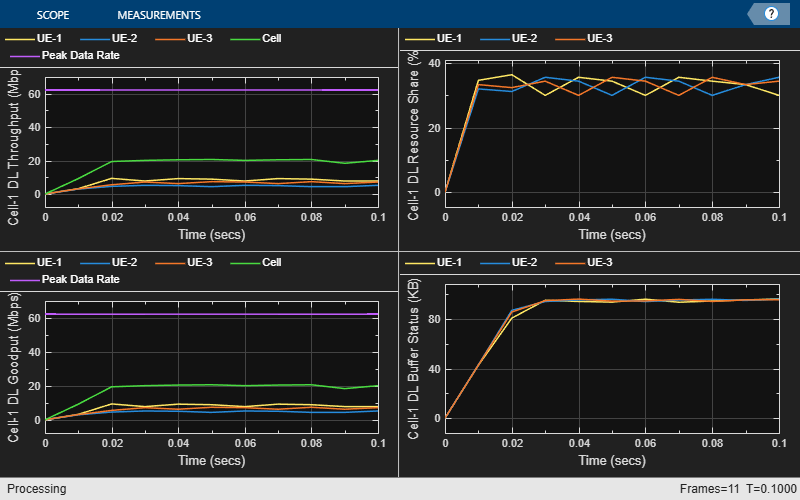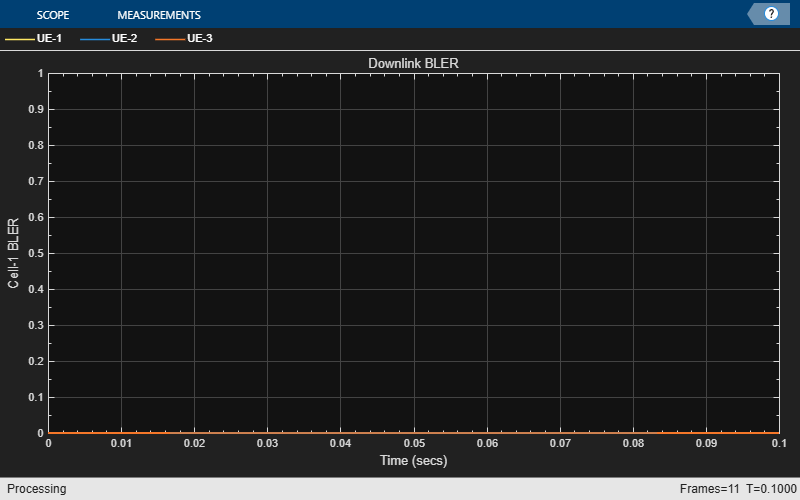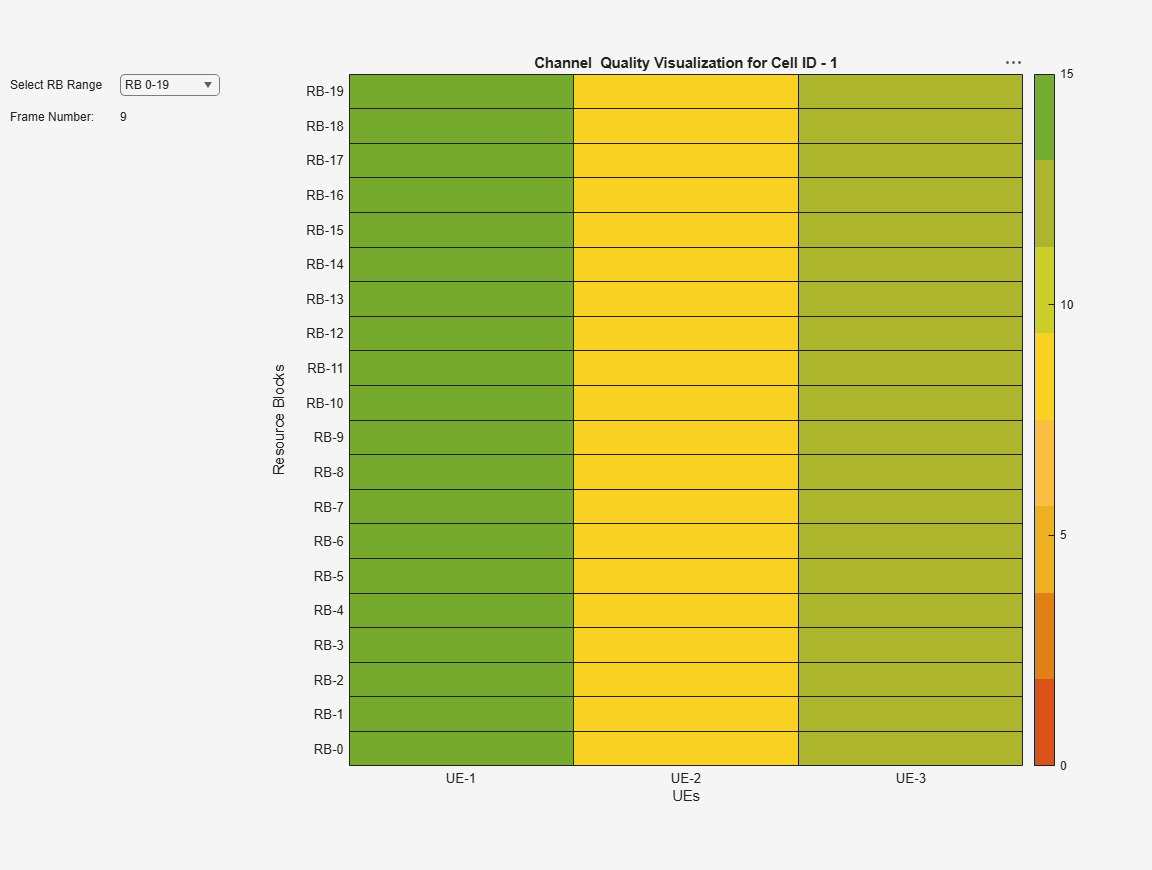Plot Tx beamforming pattern. This plot displays the selected CSI-RS beam for a UE with highest L1-RSRP. Each beam is given a unique beam index across the SSBs.

```if enableBeamforming hPlotBeamformingPattern(simParameters, gNB, beamIndices); end```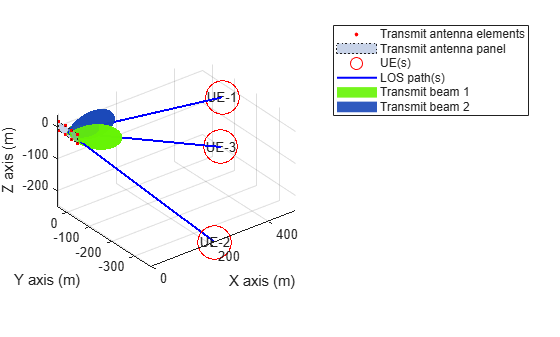Get the simulation metrics and save it in a MAT-file. The simulation metrics are saved in a MAT-file with the file name as `simulationMetricsFile`.

```metrics = getMetrics(metricsVisualizer); save(simulationMetricsFile,'metrics');```

At the end of the simulation, the achieved value for system performance indicator is compared to their theoretical peak values (considering zero overheads). Performance indicators displayed are achieved data rate (DL), achieved spectral efficiency (DL), and BLER observed for UEs (DL). The peak values are calculated as per 3GPP TR 37.910. The number of layers used for the peak DL data rate calculation is taken as the average value of the maximum layers possible for each UE in the respective direction. The maximum number of DL layers possible for a UE is minimum of its Rx antennas and gNB's Tx antennas.

`displayPerformanceIndicators(metricsVisualizer);`
```Peak DL Throughput: 62.21 Mbps. Achieved Cell DL Throughput: 18.96 Mbps Achieved DL Throughput for each UE: [7.98 4.63 6.35] Achieved Cell DL Goodput: 18.96 Mbps Achieved DL Goodput for each UE: [7.98 4.63 6.35] Peak DL spectral efficiency: 12.44 bits/s/Hz. Achieved DL spectral efficiency for cell: 3.79 bits/s/Hz Block error rate for each UE in the downlink direction: [0 0 0] ```

The simulation results show the performance metrics with DL beamforming.

### Simulation Visualization

The five types of run-time visualization shown are:

### Results With Beamforming Disabled

These are the simulation results when DL beamforming is disabled. You can observe the network performance and CQI index for each UE.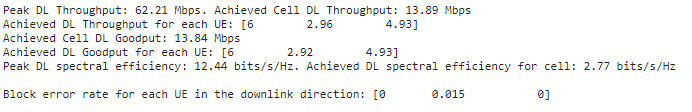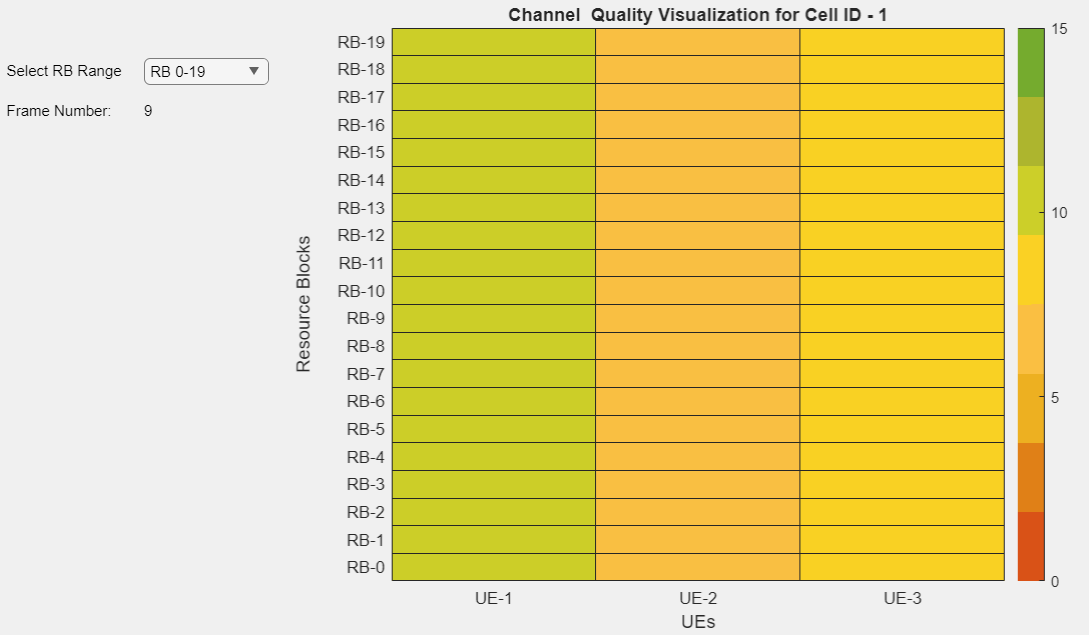For details of simulation logs, see the 'Simulation Logs' section in the NR Cell Performance Evaluation with Physical Layer Integration example.

```if enableTraces simulationLogs = cell(1,1); if simParameters.DuplexMode == 0 % FDD logInfo = struct('DLTimeStepLogs',[],'SchedulingAssignmentLogs',[],'BLERLogs',[],'AvgBLERLogs',[]); [logInfo.DLTimeStepLogs,~] = getSchedulingLogs(simSchedulingLogger); else % TDD logInfo = struct('TimeStepLogs',[],'SchedulingAssignmentLogs',[], ... 'BLERLogs',[],'AvgBLERLogs',[]); logInfo.TimeStepLogs = getSchedulingLogs(simSchedulingLogger); end % BLER logs [logInfo.BLERLogs,logInfo.AvgBLERLogs] = getBLERLogs(simPhyLogger); % Scheduling assignments log logInfo.SchedulingAssignmentLogs = getGrantLogs(simSchedulingLogger); simulationLogs{1} = logInfo; % Save simulation parameters in a MAT-file save(parametersLogFile,'simParameters'); % Save simulation logs in a MAT-file save(simulationLogFile,'simulationLogs'); end```

You can run the script NRPostSimVisualization to get a post-simulation visualization of logs. For more details about the options to run this script, refer to the NR Cell Performance Evaluation with Physical Layer Integration example.

### Further Exploration

You can use this example to further explore custom scheduling.

#### Custom scheduling

You can modify the existing scheduling strategy to implement a custom one. Plug In Custom Scheduler in System-Level Simulation example explains how to create a custom scheduling strategy and plug it into system-level simulation. MIMO configuration appends more fields to the scheduling assignment structure. Populate the fields of scheduling assignments with values for precoding matrix, number of layers, beamforming table index as per your custom scheduling strategy. The beamforming table index corresponds to the columns in the table 'simParameters.BeamWeightTable'. For more information about the information fields of a scheduling assignment, see the description of the `scheduleDLResourcesSlot` and `scheduleULResourcesSlot` functions in the hNRScheduler.m helper file.

### Local functions

```function ssbIndex = computeSSBToUE(simParameters,ssbTxAngles,ssbSweepRange,ueRelPosition) % SSBINDEX = computeSSBtoUE(SIMPARAMETERS,SSBTXANGLES,SSBSWEEPRANGE,UERELPOSITION) % computes an SSB to UE based on SSBTXANGLES and SSBSWEEPRANGE. If a UE % falls within the sweep range of an SSB then the corresponding SSBINDEX % is assigned. If a UE does not fall under any of the SSBs then the % function approximates UE to its nearest SSB. ssbIndex = zeros(1,simParameters.NumUEs); for i=1:simParameters.NumUEs if (ueRelPosition(i,2) > (ssbTxAngles(1) - ssbSweepRange/2)) && ... (ueRelPosition(i,2) <= (ssbTxAngles(1) + ssbSweepRange/2)) ssbIndex(i) = 1; elseif (ueRelPosition(i,2) > (ssbTxAngles(2) - ssbSweepRange/2)) && ... (ueRelPosition(i,2) <= (ssbTxAngles(2) + ssbSweepRange/2)) ssbIndex(i) = 2; elseif (ueRelPosition(i,2) > (ssbTxAngles(3) - ssbSweepRange/2)) && ... (ueRelPosition(i,2) <= (ssbTxAngles(3) + ssbSweepRange/2)) ssbIndex(i) = 3; elseif (ueRelPosition(i,2) > (ssbTxAngles(4) - ssbSweepRange/2)) && ... (ueRelPosition(i,2) <= (ssbTxAngles(4) + ssbSweepRange/2)) ssbIndex(i) = 4; else % Approximate UE to the nearest SSB % Initialize an array to store the difference between the UE azimuth and % SSB azimuth beam sweep angles angleDiff = zeros(1,length(ssbTxAngles)); for angleIdx = 1: length(ssbTxAngles) angleDiff(angleIdx) = abs(ssbTxAngles(angleIdx) - ueRelPosition(i,2)); end % Minimum azimuth angle difference [~,idx] = min(angleDiff); ssbIndex(i) = idx; end end end```

 3GPP TS 38.214. “NR; Physical layer procedures for data.” 3rd Generation Partnership Project; Technical Specification Group Radio Access Network.

 3GPP TS 38.321. “NR; Medium Access Control (MAC) protocol specification.” 3rd Generation Partnership Project; Technical Specification Group Radio Access Network.

 3GPP TS 38.322. “NR; Radio Link Control (RLC) protocol specification.” 3rd Generation Partnership Project; Technical Specification Group Radio Access Network.

 3GPP TR 38.901. “Study on channel model for frequencies from 0.5 to 100 GHz.” 3rd Generation Partnership Project; Technical Specification Group Radio Access Network.